# Exponentiation + square (second power, quadratic) - math problems

#### Number of problems found: 47

• Binomials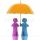To binomial ? add a number to the resulting trinomial be square of binomial.
• Roots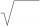Calculate the square root of these numbers:
• Unknown number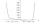I think number. If subtract from the twelfth square the ninth square I get a number 27 times greater than the intended number. What is this unknown number?
• Proof I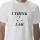When added to the product of two consecutive integers larger one, we get square larger one. Is this true or not?
• Unknown number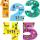Determine the unknown number, which double of its fourth square is equal the fifth its square.
• Powers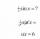Is true for any number a,b,c equality:? ?
• Exp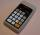If ?, then n is:
• PowerNumber ?. Find the value of x.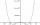By introducing a new variable solve biquadratic equation: ?
• Digits A, B, CFor the various digits A, B, C is true: the square root of the BC is equal to the A and sum B+C is equal to A. Calculate A + 2B + 3C. (BC is a two-digit number, not a product).
• Geometric sequenceIn the geometric sequence is a4 = 20 a9 = -160. Calculate the first member a1 and quotient q.
• What isWhat is the annual percentage increase in the city when the population has tripled in 20 years?
• Cube diagonalDetermine the length of the cube diagonal with edge 37 mm.
• Find the 2Find the term independent of x in the expansion of (4x3+1/2x)8
• Substitution method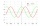Solve goniometric equation: sin4 θ - 1/cos2 θ=cos2 θ - 2
• Simplest form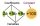Find the simplest form of the following expression: 3 to the 2nd power - 1/4 to the 2nd power
• SaleIf the product twice price cut by 25%, what percentage was price cut in total?
• Geometric sequence 3In geometric sequence is a8 = 312500; a11= 39062500; sn=1953124. Calculate the first item a1, quotient q and n - number of members by their sum s_n.
• CubeOne cube has edge increased 5 times. How many times will larger its surface area and volume?
• Cube 6Volume of the cube is 216 cm3, calculate its surface area.

Do you have an interesting mathematical word problem that you can't solve it? Submit a math problem, and we can try to solve it.

We will send a solution to your e-mail address. Solved examples are also published here. Please enter the e-mail correctly and check whether you don't have a full mailbox.

Please do not submit problems from current active competitions such as Mathematical Olympiad, correspondence seminars etc...

Exponentiation - math word problems. Square (second power, quadratic) - math word problems.